# Parity of zero

﻿
Parity of zero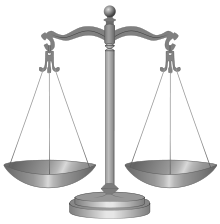Zero objects, divided into two equal groups

Zero is an even number. In other words, its parity—the quality of an integer being even or odd—is even. Zero fits the definition of "even number": it is an integer multiple of 2, namely 0 × 2. As a result, zero shares all the properties that characterize even numbers: 0 is evenly divisible by 2, 0 is surrounded on both sides by odd numbers, 0 is the sum of an integer with itself, and a set of 0 objects can be split into two equal sets.

Since definitions can change, another approach is to set them aside and consider how zero fits into the patterns formed by other even numbers. The parity rules of arithmetic, such as even − even = even, require 0 to be even. Zero is the identity element of the group of even integers, and it is the starting case from which other even natural numbers are recursively generated. Applications of this recursion from graph theory to computational geometry rely on zero being even. Not only is 0 divisible by 2, it is divisible by every integer. In the binary numeral system used by computers, it is especially relevant that 0 is divisible by every power of 2; in this sense, 0 is the "most even" number of all.

Among the general public, the parity of zero can be a source of confusion. In reaction time experiments, most people are slower to label 0 as even than 2, 4, 6, or 8. In schools, both students and teachers often hold misconceptions that zero is odd, or both even and odd, or neither. Researchers in mathematics education propose that these misconceptions can become learning opportunities. Studying equations like 0 × 2 = 0 can address students' doubts about calling 0 a number and using it in arithmetic. Discussing the issue in class can spark debates between students, during which they encounter basic principles of mathematical reasoning, such as the importance of definitions. Understanding zero is one goal, but there is also a wider lesson. Evaluating the parity of this exceptional number is an early example of a pervasive theme in mathematics: the abstraction of a familiar concept to an unfamiliar setting.

## Why zero is even

It is easy to directly prove that zero is even:

• A number is called even if it is an integer multiple of 2. Zero is an integer multiple of 2, namely 0 × 2, so zero is even.

This proof starts with a standard definition of "even number". It is also possible to explain why zero is even without referring to formal definitions. The following explanations make sense of the idea that zero is even in terms of fundamental number concepts. From this foundation, one can provide a rationale for the definition itself—and its applicability to zero.

### Basic explanations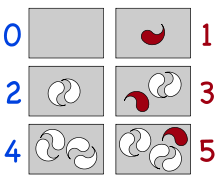The box with 0 objects has no red object left over.

A basic usage for numbers is counting. Given a set of objects, one uses a number to describe how many objects are in the set. Zero is the count of no objects; in more formal terms, it is the number of objects in the empty set. The concept of parity is used in making groups of two objects. If the objects in a set can be marked off into groups of two, with none left over, then the number of objects is even. If an object is left over, then the number of objects is odd.

The empty set contains zero groups of two, and no object is left over from this grouping, so zero is even. Although it is difficult to depict zero groups of two, or to draw attention to the nonexistence of a leftover object, this conception of the evenness of zero can be illustrated by comparing the empty set with other sets, as in the diagram on the right.

The number line provides a more uniform way of depicting numbers, including positive numbers, negative numbers, and zero. When even and odd numbers are distinguished visually, their pattern becomes obvious:

The even and odd numbers alternate. Starting at any even number, counting up or down by twos reaches the other even numbers, and there is no reason to skip over zero.

Parity can be approached in a more formal way using arithmetic expressions. Every integer is either of the form (2 × ▢) + 0 or (2 × ▢) + 1; the former numbers are even and the latter are odd. For example, 1 is odd because 1 = (2 × 0) + 1, and 0 is even because 0 = (2 × 0) + 0. Making a table of these facts then reinforces the number line picture above.

### Defining parity

The precise definition of any mathematical term, such as "even" meaning "integer multiple of two", is ultimately a convention. Unlike "even", some mathematical terms are purposefully constructed to exclude trivial or degenerate cases. Prime numbers are a famous example. The definition of "prime number" has historically shifted from "positive integer with at most 2 factors" to "positive integer with exactly 2 factors", with the effect that 1 is no longer considered prime. Most authors rationalize this shift by observing that the modern definition more naturally suits mathematical theorems that concern the primes. For example, the fundamental theorem of arithmetic is easier to state when 1 is not considered prime.

It would be possible to similarly redefine the term "even" in a way that no longer includes zero. However, in this case, the new definition would make it more difficult to state theorems concerning the even numbers. Already the effect can be seen in the algebraic rules governing even and odd numbers. The most relevant rules concern addition, subtraction, and multiplication:

• even ± even = even
• odd ± odd = even
• even × integer = even

Inserting appropriate values into the left sides of these rules, one can produce 0 on the right sides:

• 2 − 2 = 0
• −3 + 3 = 0
• 4 × 0 = 0

The above rules would therefore be incorrect if zero were not even. At best they would have to be modified. For example, one test study guide asserts that even numbers are characterized as integer multiples of two, but zero is "neither even nor odd". Accordingly, the guide's rules for even and odd numbers contain exceptions:

• even ± even = even (or zero)
• odd ± odd = even (or zero)
• even × nonzero integer = even

Making an exception for zero in the definition of evenness forces one to make such exceptions in the rules for even numbers. From another perspective, taking the rules obeyed by positive even numbers, and requiring that they continue to hold for integers, forces the usual definition and the evenness of zero.

## Mathematical contexts

Countless results in number theory invoke the fundamental theorem of arithmetic and the algebraic properties of even numbers, so the above choices have far-reaching consequences. For example, the fact that numbers have unique factorizations means that one can determine whether a number has an even or odd number of distinct prime factors. Since 1 is not prime, nor does it have prime factors, it is a product of 0 distinct primes; since 0 is an even number, 1 has an even number of distinct prime factors. This implies that the Möbius function takes the value μ(1) = 1, which is necessary for it to be a multiplicative function and for the Möbius inversion formula to work, and affects the exact value of the Mertens function everywhere.

### Not being odd

The observation that zero is not odd is sometimes directly applied in a mathematical argument. If an unknown number is proven to be odd, then it cannot be zero. This apparently trivial observation can provide a convenient and revealing proof explaining why a number is nonzero.

A classic result of graph theory states that a graph of odd order always has at least one even vertex. (Already this statement requires zero to be even: the empty graph has an even order, and an isolated vertex is even.) In order to prove the statement, it is actually easier to prove a stronger result: any odd-order graph has an odd number of even vertices. The appearance of this odd number is explained by a still more general result, known as the handshaking lemma: any graph has an even number of vertices of odd degree. Finally, the even number of odd vertices is naturally explained by the degree sum formula.

Sperner's lemma is a more advanced application of the same strategy. Rather than prove that there exists a completely labeled subsimplex by a direct construction, it is more convenient to prove that there exist an odd number of such subsimplices through an induction argument. A still stronger statement of the lemma then explains why this number is odd: it naturally breaks down as (n + 1) + n when one segregates colorings by orientation.

### Even-odd alternation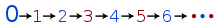Recursive definition of natural number parity

The fact that zero is even, together with the fact that even and odd numbers alternate, is enough to determine the parity of every other natural number. This idea can be formalized into a recursive definition of the set of even natural numbers:

• 0 is even.
• (n + 1) is even if and only if n is not even.

This definition has the conceptual advantage of relying only on the minimal foundations of the natural numbers: the existence of 0 and of successors. As such, it is useful for computer logic systems such as the Isabelle theorem prover. With this definition, the evenness of zero is not a theorem but an axiom. Indeed, "zero is an even number" may be interpreted as one of the Peano axioms, of which the even natural numbers are a model. A similar construction extends the definition of parity to transfinite ordinal numbers: every limit ordinal is even, including zero, and successors of even ordinals are odd.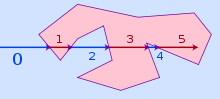Point in polygon test

The classic point in polygon test from computational geometry applies the above ideas. To determine if a point lies within a polygon, one casts a ray from infinity to the point and counts the number of times the ray crosses the edge of polygon. The crossing number is even if and only if the point is outside the polygon. This algorithm works because if the ray never crosses the polygon, then its crossing number is zero, which is even, and the point is outside. Every time the ray does cross the polygon, the crossing number switches between even and odd, and the point at its tip switches between outside and inside.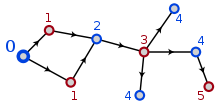Constructing a bipartition

Another application comes from the field of graph theory. A graph whose vertices are split into two groups, such that two vertices from the same group are never adjacent, is called a bipartite graph. If a (connected) graph has no odd cycles, then an explicit bipartition can be constructed by choosing a base vertex v and coloring every vertex black or white, depending on whether its distance from v is even or odd. Since the distance between v and itself is 0, and 0 is even, the base vertex is colored the opposite color as its neighbors, which lie at a distance of 1.

### Algebraic patterns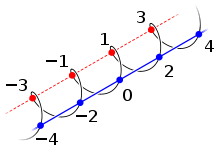2Z (blue) as subgroup of Z

The evenness of zero appears in the structured context of abstract algebra. The fact that the additive identity (zero) is even, together with the evenness of sums and additive inverses of even numbers and the associativity of addition, means that the even integers form a group. Moreover, the group of even integers under addition is a subgroup of the group of all integers; this is an elementary example of the subgroup concept. The earlier observation that the rule "even − even = even" forces 0 to be even is part of a general pattern: any nonempty subset of an additive group that is closed under subtraction must be a subgroup, and in particular, must contain the identity.

Since the even integers form a subgroup of the integers, they partition the integers into cosets. These cosets may be described as the equivalence classes of the following equivalence relation: x ~ y if (xy) is even. Here, the evenness of zero is directly manifested as the reflexivity of the binary relation ~. There are only two cosets of this subgroup—the even and odd numbers—and it can be used as a template for subgroups with index 2 in other groups as well. A well-known example is the alternating group, a subgroup of the symmetric group on n letters. The elements of the alternating group, called even permutations, are the products of even numbers of transpositions. The identity map, an empty product of no transpositions, is an even permutation since zero is even; it is the identity element of the group.

Adding in the rule "even × integer = even" means that the even numbers form an ideal in the ring of integers, and the above equivalence relation can be described as equivalence modulo this ideal. In particular, even integers are exactly those integers k where k ≡ 0 (mod 2). This formulation is useful for investigating integer zeroes of polynomials.

### Degrees of evenness

There is a sense in which some multiples of 2 are "more even" than others. The ancient Greeks already categorized the even numbers as singly and doubly even; 0 is doubly even because it is a multiple of 4, so it can be divided by 2 twice. Indeed, 0 has the unique property of being divisible by every power of 2, so it surpasses all other numbers in "evenness".

One consequence of this fact appears in computer algorithms such as the Cooley–Tukey FFT, in which numbers appear in bit-reversed order. This ordering has the property that the farther to the left the first 1 occurs in a number's binary expansion, or the more times it is divisible by 2, the sooner it appears. Zero's bit reversal is still zero; it can be divided by 2 any number of times, and its binary expansion does not contain any 1s, so it always comes first. The illustration on the right depicts the evenness of the integers from +64 to −64.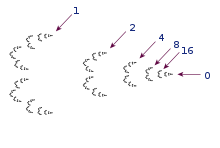The 2-adic integers (black points). Arrows point to 0 and powers of 2.

It is clear that 0 is divisible by 2 more times than any other number, but one runs into trouble when trying to quantify exactly how many times that is. For any nonzero integer n, one may define the 2-adic order of n: an integer which can be described as the number of times n is divisible by 2, or the exponent of the largest power of 2 that divides n, or the multiplicity of 2 in the prime factorization of n. But none of these descriptions works for 0; no matter how many times 0 is halved, it can still be halved again. Rather, the usual convention is to set the 2-order of 0 to be infinity as a special case. This convention is not peculiar to the 2-order; it is one of the axioms of an additive valuation in higher algebra.

The powers of two—1, 2, 4, 8, ...—form a simple sequence of increasingly even numbers. There are mathematically interesting ways to force such sequences to actually converge to zero, including the construction of the 2-adic numbers.

## History

It is difficult to say when in the history of mathematics the first person examined the parity of zero; there is no well-documented answer. What is certain is that even and odd numbers were known before the number zero was introduced. Indeed, this historical development parallels children's conceptual development. The algebraic properties of 0, such as the rule 0 × n = 0, were first systematically explored by Indian mathematicians such as Brahmagupta in the 7th century—relatively late in comparison to the early history of number theory.

Ancient Greek mathematicians generally considered 2 to be the first even number and 3 the first odd number, and some did not even recognize 2 as even. The number 1 was not truly a number, but a component of all other numbers; as such it had to be both even and odd, and therefore neither truly even nor truly odd. This dual role for 1 was a source of metaphysical discomfort; one historian asserts that the Greeks could have avoided the issue had they known about 0.

## Education

The subject of the parity of zero is often treated within the first two or three years of primary education, as the concept of even and odd numbers is introduced and developed.

### Students' knowledge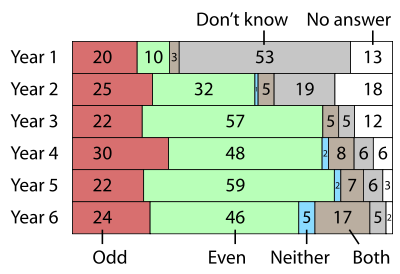% responses over time

The chart on the right depicts children's beliefs about the parity of zero, as they progress from Year 1 to Year 6. The data are from Len Frobisher, who conducted a pair of surveys of UK schoolchildren. Frobisher was interested in how knowledge of single-digit parity translates to knowledge of multiple-digit parity, and zero figures prominently in the results.

In a preliminary survey of nearly 400 seven-year-olds, 45% chose even over odd when asked the parity of zero. A follow-up investigation offered more choices: neither, both, and don't know. This time the number of children in the same age range identifying zero as even dropped to 32%. Success in deciding that zero is even initially shoots up and then levels off at around 50% in Years 3 to 6. For comparison, the easiest task, identifying the parity of a single digit, levels off at about 85% success.

In interviews, Frobisher elicited the students' reasoning. One fifth-year decided that 0 was even because it was found on the 2 times table. A couple fourth-years realized that zero can be split into equal parts: "no one gets owt if it's shared out." Another fourth-year reasoned "1 is odd and if I go down it's even." The interviews also revealed the misconceptions behind incorrect responses. A second-year was "quite convinced" that zero was odd, on the basis that "it is the first number you count". A fourth-year referred to 0 as "none" and thought that it was neither odd nor even, since "it's not a number".

More in-depth investigations were conducted by Esther Levenson, Pessia Tsamir, and Dina Tirosh, who interviewed a pair of sixth-grade students who were performing highly in their mathematics class. One student preferred deductive explanations of mathematical claims, while the other preferred practical examples. Both students initially thought that 0 was neither even nor odd, for different reasons. Levenson et al.'s report in the Journal of Mathematical Behavior details the students' reasoning; one of the themes is that their beliefs about the parity of zero are consistent with their concepts of zero and division.

### Teachers' knowledge

Researchers of mathematics education at the University of Michigan used the true-or-false prompt "0 is an even number", among many similar questions, in a 2000–2004 study of 700 primary teachers in the United States. For them the question exemplifies "common knowledge ... that any well-educated adult should have", and it is "ideologically neutral" in that the answer does not vary between traditional and reform mathematics. Overall performance in the study significantly predicted improvements in students' standardized test scores after taking the teachers' classes.

It is uncertain how many teachers harbor misconceptions about zero. The Michigan study did not publish data for individual questions. One report comes from Betty Lichtenberg, who wrote an article titled "Zero is an even number" in the journal The Arithmetic Teacher in 1972. Lichtenberg, an associate professor of mathematics education at the University of South Florida, draws on her experience with a course she and her colleagues taught on methods for teaching arithmetic. She reports that several sections of prospective elementary school teachers were given a true-or-false test including the item "Zero is an even number." They found it to be a "tricky question", and about two thirds answered "False".

The literature contains a couple data points concerning teachers' attitudes about students' attitudes. The National Council of Teachers of Mathematics's Principles and Standards for School Mathematics records a first grader's argument that zero is an even number: "If zero were odd, then 0 and 1 would be two odd numbers in a row. Even and odd numbers alternate. So 0 must be even..." In a survey of 10 college students preparing to teach mathematics, none of them thought that the argument sufficed as a mathematical proof. When they were told that a first grader had written the argument, most agreed that it was acceptable reasoning for that age level.

### Group discussions

Often students will independently ask if zero is even; the Israel National Mathematics Curriculum reminds first grade teachers that zero is even, but advises that it is unnecessary to mention this unless the class brings it up. In one study, Annie Keith observed a class of 15 second grade students, who convinced each other that zero was an even number based on even-odd alternation and on the possibility of splitting a group of zero things in two equal groups.

Deborah Loewenberg Ball, a researcher specializing in mathematics instruction, has extensively analyzed an episode that occurred in her public school classroom of 22 third graders during the 1989–1990 school year. Ball asked the class for comments about even and odd numbers and zero, which they had just been discussing with a group of fourth-graders, and over the next six minutes the students made a variety of claims about the parity of zero, the rules for even numbers, and how mathematics is done. The claims about zero alone take many forms: Zero is not even or odd; Zero could be even; Zero is not odd; Zero has to be an even; Zero is not an even number; Zero is always going to be an even number; Zero is not always going to be an even number; Zero is even; Zero is special. Much of the discussion hinges around one student's comment that "even numbers make even numbers", but that zero is "special"; students seem to advance competing definitions of "even", all the while bringing up examples and counterexamples to each other's claims.

Ball later asked her students to reflect on this "particularly long and confusing discussion on even and odd numbers". One student commented that hearing other ideas had helped her understanding, and she now believed for the first time that zero was even. At the same time, another student had originally thought zero to be even but "got sort of mixed up" and was unsure whom to agree with. Ball finds it significant that the latter student expressed a desire to listen further to the discussion: in this sense, both students have learned something valuable about their own learning process. On another occasion, during a discussion on fractions, Ball asked the class whether voting is a good way to prove what is true in mathematics. One of the students returned to her experience of the discussion on zero, concluding that voting was ineffective in comparison to investigating patterns.

### Implications for instruction

Mathematically, no further proof is required, but more explanation is needed in the context of education. One issue concerns the foundations of the proof: the concise definition of "even" given above is not always appropriate. A student in the first years of primary education may not yet have learned what "integer" or "multiple" means, much less how to multiply with 0. Additionally, stating a definition of parity for all integers can seem like an arbitrary conceptual shortcut if the only even numbers investigated so far are 2, 4, 6, 8, and higher. It can help to acknowledge that as the number concept is extended from positive integers to include zero and negative integers, so too number properties such as parity are extended in a nontrivial way.

Age-appropriate explanations that zero is even, then, return to the concrete interpretation of parity in terms of paired objects, or they emphasize the even-odd alternation between numbers. Meanwhile misconceptions about 0 must be combated, such as the belief that 0 means nothing and has no properties.

## Numerical cognition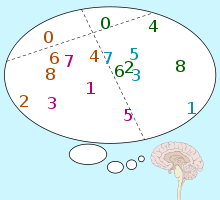Smallest space analysis of reaction times, showing separation of 0

Adults who do believe that zero is even can nevertheless feel unfamiliar or uncomfortable with the fact, enough to measurably slow them down in a reaction time experiment. For an experiment designed to investigate the task of parity determination, a numeral or a number word is flashed to the subject on a monitor, and a computer records the time it takes the subject to identify the number as odd or even by striking an appropriate button, such as a Morse key. Stanislas Dehaene, a pioneer in the field of numerical cognition, led a series of such experiments in the early 1990s. They showed that 0 was slower to process than other even numbers. Some variations of the experiment found delays as long as 60 milliseconds or about 10% of the average reaction time—a small difference but a significant one.

Dehaene's experiments were not designed specifically to investigate 0, but to compare competing models of how parity information is processed and extracted. The most specific extraction model, the mental calculation hypothesis, suggests that reactions to 0 should be fast: 0 is a small number, and it is easy to calculate 0 × 2 = 0. (Subjects are known to compute and name the result of multiplication by zero faster than multiplication of nonzero numbers, although they are slower to verify proposed results like 2 × 0 = 0.) The results of the experiments suggested that something quite different was happening: parity information was apparently being recalled from memory along with a cluster of related properties, such as being prime or a power of two. Both the sequence of powers of two and the sequence of positive evens 2, 4, 6, 8, ... are well-distinguished mental categories whose members are prototypically even. Zero belongs to neither list, hence the slower responses.

Repeated experiments have shown a delay at zero for subjects from a variety of national and linguistic backgrounds, representing both left to right and right to left writing systems; almost all right-handed; from 17–53 years of age; confronted with number names in numeral form, spelled out, and spelled in a mirror image. Dehaene's group did find one differentiating factor: mathematical expertise. In one of their experiments, students in the École Normale Supérieure were divided into two groups: those in literary studies and those studying mathematics, physics, or biology. The slowing at 0 was "essentially found in the [literary] group", and in fact, "before the experiment, some L subjects were unsure whether 0 was odd or even and had to be reminded of the mathematical definition".

This strong dependence on familiarity again undermines the mental calculation hypothesis. The effect also suggests that it is inappropriate to include zero in experiments where even and odd numbers are compared as a group. As one study puts it, "Most researchers seem to agree that zero is not a typical even number and should not be investigated as part of the mental number line."

## Everyday contexts

Some of the contexts where the parity of zero makes an appearance are purely rhetorical:

• It provides material for Internet message boards and ask-the-expert websites.
• Linguist Joseph Grimes muses that asking "Is zero an even number?" to married couples is a good way to get them to disagree.
• Social theorist Anthony Wilden proposes that all rules have exceptions, giving an example: "Whole numbers are either odd or even, but zero is neither one nor the other."
• Columnist Tony Snow once wrote, "Question: Name one thing beneath Bill Clinton's dignity. Answer: This is a trick question, like asking whether zero is odd or even. It has no known answer." The attack on Clinton was to be expected; three readers who protested the comparison were more concerned with the mathematical error.
• Around the turn of the third millennium, media outlets noted a pair of unusual milestones: "11/19/1999" was the last calendar date composed of all odd digits that would occur for a very long time, and that "02/02/2000" was the first all-even date to occur in a very long time. Since these results make use of 0 being even, some readers disagreed with the idea.

There are also situations where calling zero even, or not, has consequences:

One third-party study guide for the GMAT states that 0 is not even, but the test's authors publish an official study guide that explicitly includes 0 in the even numbers. The correct answers to some of the GMAT's "data sufficiency" questions require that the usual rules for even numbers, such as n being even if (n + 2) is even, hold without exception for 0. The GRE also stipulates that 0 is even. Generally, on standardized tests, if a question asks about the behavior of even numbers, it might be necessary to keep in mind that zero is even.

The nominal evenness of zero is relevant to odd-even rationing systems. Cars might be allowed to drive or to purchase gasoline on alternate days, according to the parity of the last digit in their license plates. Half of the numbers in a given range end in 0, 2, 4, 6, 8 and the other half in 1, 3, 5, 7, 9, so it makes sense to include 0 with the other even numbers. The relevant legislation sometimes stipulates that zero is even to avoid confusion. In fact, an odd-even restriction on driving in 1977 Paris did lead to confusion when the rules were unclear. On an odd-only day, the police avoided fining drivers whose plates ended in 0, because they did not know whether 0 was even.

In other situations, it can make sense to separate 0 from the other even numbers. On U.S. Navy vessels, even-numbered compartments are found on the port side, but zero is reserved for compartments that intersect the centerline. That is, the numbers read ...6420135... from port to starboard.

In the game of roulette, the casino has an interest in making sure that less than half of the numbers are counted as even. Thus the number 0 does not count as even or odd; a bet placed on either even or odd does not win if the ball falls on "0" or "00". The exact result depends on local rules, but the overall effect is to give the house an edge on "even money" bets. Similarly, the parity of zero can affect payoffs in prop bets when the outcome depends on whether some randomized number is odd or even, and it turns out to be zero. One bookmaker offers a "cricket roulette" in which a batsman who is dismissed for a duck wins for the bank.

The game of "odds and evens" is also affected: if both players cast zero fingers, who wins? Generally zero is counted as even. In fact, playing this game has been suggested as a way of introducing children to the concept that 0 is divisible by 2.

Wikimedia Foundation. 2010.

### Look at other dictionaries:

• Parity (mathematics) — In mathematics, the parity of an object states whether it is even or odd. This concept begins with integers. An even number is an integer that is evenly divisible by 2, i.e., divisible by 2 without remainder; an odd number is an integer that is… …   Wikipedia

• Parity (physics) — Flavour in particle physics Flavour quantum numbers: Isospin: I or I3 Charm: C Strangeness: S Topness: T Bottomness: B′ Related quantum numbers: Baryon number: B Lepton number: L Weak isospin: T or T3 Electric charge: Q …   Wikipedia

• parity —    In communications, a simple form of error checking that uses an extra or redundant bit after the data bits but before the stop bit or bits. Parity may be set as follows:    • Odd    Indicates that the sum of all the 1 bit is set to zero; if it …   Dictionary of networking

• Parity-check matrix — In coding theory, a parity check matrix of a linear block code C is a generator matrix of the dual code. As such, a codeword c is in C if and only if the matrix vector product Hc=0. The rows of a parity check matrix are parity checks on the… …   Wikipedia

• Parity of a permutation — Permutations of 4 elements Odd permutations have a green or orange background. The numbers in the right column are the inversion numbers (sequence …   Wikipedia

• Parity flag — In computer processors the parity flag indicates if the number of set bits is odd or even in the binary representation of the result of the last operation. It is normally a single bit in a processor status register.For example, assume a machine… …   Wikipedia

• Parity P — In computational complexity theory, the complexity class {oplus}mathbf{P} (pronounced parity P ) is the class of decision problems solvable by a nondeterministic Turing machine in polynomial time, where the acceptance condition is that the number …   Wikipedia

• Parité de zéro — Zéro objets, divisés en deux groupes égaux. Zéro est un nombre pair, étant donné qu il remplit la définition d un nombre pair : être un multiple de deux. Par conséquent, zéro partage toutes les propriétés qui découlent de cette… …   Wikipédia en Français

• Evenness of zero — The number 0 is even. There are several ways to determine whether an integer is even or odd, all of which indicate that 0 is an even number: it is a multiple of 2, it is evenly divisible by 2, it is surrounded on both sides by odd integers, and… …   Wikipedia

• R-parity — is a concept in particle physics. In the supersymmetric extension of the Standard Model, baryon number and lepton number are no longer conserved by all of the renormalizable couplings in the theory. Since baryon number and lepton number… …   Wikipedia